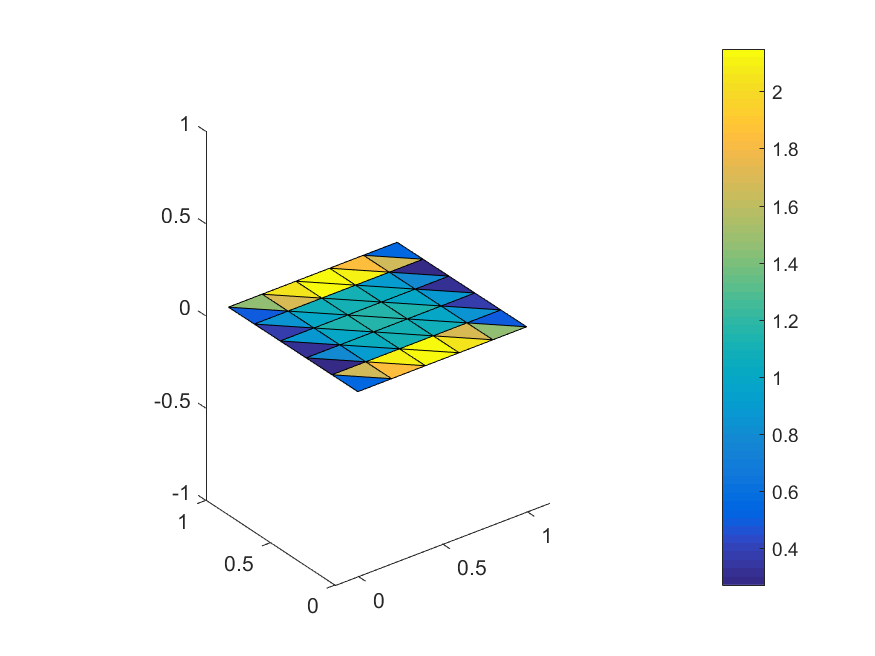# Tutorial

In this tutorial we will go through the steps required for the formulation and the solution of the scattering of a time harmonic electromagnetic wave by a rectangular plate by means of the solution of the electric field integral equation.

## Building the geometry

The sibling package CompScienceMeshes provides data structures and algorithms for working with simplical meshes in computational science. We will use it to create the geometry:

using CompScienceMeshes, BEAST
o, x, y, z = euclidianbasis(3)

h = 0.2
Γ = meshrectangle(1.0, 1.0, h)
@show numvertices(Γ)
@show numcells(Γ)

numvertices(Γ) = 36
numcells(Γ) = 50


Next, we create the finite element space of Raviart-Thomas aka Rao-Wilton-Glisson functions subordinate to the triangulation Γ constructed above:

X = raviartthomas(Γ)


The scattering problem is defined by specifying the single layer operator and the functional acting as excitation. Here, the plate is illuminated by a plane wave. The actual excitation is the tangential trace of this electric field. This trace be constructed easily by using the symbolic normal vector field n defined as part of the BEAST package.

κ = 1.0
E = Maxwell3D.planewave(direction=ẑ, polarization=x̂, wavenumber=κ)
e = (n × E) × n


The single layer potential is also predefined by the BEAST package:

t = Maxwell3D.singlelayer(wavenumber=κ)


It corresponds to the bilinear form

Using the LinearForms package, which implements a simple form compiler for Julia (@varform), the EFIE can be defined and discretised by

@hilbertspace j
@hilbertspace k
efie = @discretise t[k,j]==e[k]  j∈X k∈X


Solving and computing the values of the induced current in the centers of the triangles of the mesh is now straightforward:

u = solve(efie)
fcr, geo = facecurrents(u,X)


The resulting current distribution can be visualised by e.g. Matlab, Paraview, Plotly,...